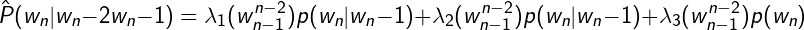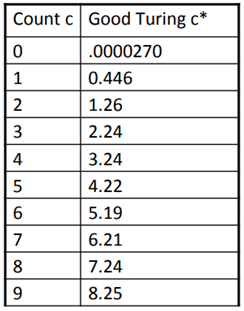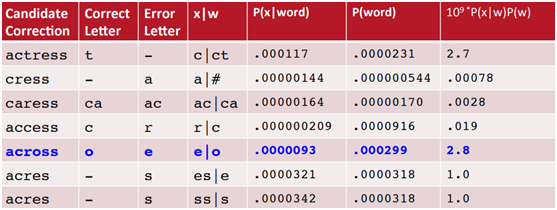## Learning NLP Perplexity and Smoothing in Python

##### In this part of Learning Python we Cover NLP Perplexity and Smoothing In Python
Written by |30-Jul-2019 | 0 Comments | 553 Views

Back-off and Interpolation:

This can be elaborated as if we have no example of a particular trigram we can instead estimate its probability by using a bigram. Similarly, if we don't have a bigram either, we can look up to unigram. This is a back off method and by interpolation always mix the probability estimates from all the ngram, weighing and combining the trigram, bigram and unigram count.

In simple linear interpolation, the technique we use is we combine different order of n-grams ranging from 1 to 4 grams for the model. Thus we calculate trigram probability together unigram, bigram, and trigram each weighted by lambda.

p̂(wn|wn-2wn-1) = λ1P(wn|wn-2wn-1)+λ2P(wn|wn-1)+λ3P(wn)

Such that the lambda’s sum to 1. In a marginally more sophisticated version of linear interpolation, each lambda weight is computed by conditioning on the context. In this way if we have accurate numbers of particular bigram we can assume the number of trigrams based on this bigram which will be a more robust method to implement so the equation can be:Both the simple interpolation and conditional winter held-out polation lambdas are learned from a held-out corpus. A held-out corpus is an additional training corpus that we use to set hyperparameters like these lambda values, by choosing the lambda values that maximize the likelihood of the held-out corpus. That is, we adjust the n-gram probabilities and then search for the lambda values that give us the highest probability of the held-out set. There are numerous approaches to find this optimal set of lambdas. The straightforward way is to use the EM algorithm, an iterative learning algorithm that converges on locally optimal lambda’s.

Good Turing:

It re-estimates probability mass assigned to n-grams with zero counts.

• Use ratio between n+1 and n-grams
• Reallocate the probability mass of n-grams ( that occurs c+1 times in the training data) to the n-grams (that occurs c time)
• based on the assumption of the binomial distribution

Why use Good Turing?

• Good Turing estimates the new things by the things we saw once.
• It tells us the probability of things we have never seen before.

Suppose we have a scenario 10 carp, 3 perch,2 whitefish, 1 trout, 1 salmon, 1 eel = 18 fish

What is the probability of catfish or bass?

Let use our estimate of things we saw once to estimate the new things can be calculated as follows:

unseen = c = 0

P* = N1/N = 3/18

Problem:

• for small k, Nk > Nk+1.
• For large k, the graph will be too jumpy.

Kneser-Ney Smoothing:If we look at the table of good Turing carefully, we can see that the good Turing c of seen values are the actual negative of some value ranging (0.7-0.8). So Kneser-ney smoothing saves ourselves some time and subtract 0.75, and this is called Absolute Discounting Interpolation.The above equation shows how to calculate Absolute discounting. Here d is the discount which can be 0.75 or some other d.

The unigram is useful to exactly when we haven’t seen the particular bigram.

Why use Kneser Ney?

A typical precedent that represents the idea of driving this technique is the recurrence of the bigram San Francisco. On the off chance that it seems a few times in a preparation corpus, the repetition of the unigram "Francisco" will likewise be high. Depending on just the unigram recurrence to foresee the frequencies of n-grams prompts skewed outcomes to be that as it may, Kneser– Ney smoothing amends this by considering the recurrence of the unigram in connection to potential words going before it.

Noisy Channel Model:

The original work of Shanon focused on finding a coding that would make information redundant enough so that the original message could be retrieved even in the presence of noise. For a memoryless channel, the second Shannon law states that a channel capacity can be determined based on mutual information:

C = max I( X ; Y )

In NLP applications, we try to restore the original input from the output of a noisy channel. As the output is given, it is constant in all cases, and its probability can be ignored:

arg max p( i | o ) = arg max p( i ) p( o | i  ) / p( o )

= arg max p( i ) p( o | i  )

## Intuition:

The intuition is to verify the signal from all of the words and check which word looks like the most.

• It is a probabilistic model.
• It was originally designed for speech recognition.
• The maximum probability will be selected.

In channel model probability it computes error probability by creating a confusion matrix. The confusion matrix allows us knowing the most probable mistake after a given letter.

## Spelling Correction:

In spelling correction, we have an incorrect string s, and a dictionary D containing exact words. We are looking for a word w element of D that is most probably the word that was changed as a result of errors. We want to find maximum probability by Bayes rule.

Suppose we have a string as following:

String = “a stellar and versatile acress whose combination of sass and glamour.”

Here the actress has wrongly spelled a word so what will be the right word. By confusion matrix we can estimate it as follows:Figure 1

Here ‘across’ is with high probability and on the 2nd number it is an actress but across don't fit best with the sentence, so we now compute bigram probability of actress and across:

Here we can conclude from the above calculation that actress is the right word.

Use Cases:

• Voice Recognition

• Dependency Parser

• Spell Checker

• Optical Character Recognition.

State of The Art System:

In state of the art system, many factors are combined to form a robust system. It includes many models to make it more efficient and accurate. Following are the models and improvement in models.

Phonetic Error Model:

• Convert misspelling to Metaphone pronunciation. Examples are the following:
1) Drop duplicate adjacent letter except for C.
2) If the word begins with ‘KN’, ‘AE’, ‘WR’, drop the first letter

• Find word whose pronunciation is 1-2 edit distance from the misspelling. By doing this, we will cut a lot of computation which has to be done otherwise.